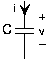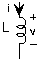# Appendix 2: Ideal Circuit Elements

$$\newcommand{\vecs}{\overset { \rightharpoonup} {\mathbf{#1}} }$$ $$\newcommand{\vecd}{\overset{-\!-\!\rightharpoonup}{\vphantom{a}\smash {#1}}}$$$$\newcommand{\id}{\mathrm{id}}$$ $$\newcommand{\Span}{\mathrm{span}}$$ $$\newcommand{\kernel}{\mathrm{null}\,}$$ $$\newcommand{\range}{\mathrm{range}\,}$$ $$\newcommand{\RealPart}{\mathrm{Re}}$$ $$\newcommand{\ImaginaryPart}{\mathrm{Im}}$$ $$\newcommand{\Argument}{\mathrm{Arg}}$$ $$\newcommand{\norm}{\| #1 \|}$$ $$\newcommand{\inner}{\langle #1, #2 \rangle}$$ $$\newcommand{\Span}{\mathrm{span}}$$ $$\newcommand{\id}{\mathrm{id}}$$ $$\newcommand{\Span}{\mathrm{span}}$$ $$\newcommand{\kernel}{\mathrm{null}\,}$$ $$\newcommand{\range}{\mathrm{range}\,}$$ $$\newcommand{\RealPart}{\mathrm{Re}}$$ $$\newcommand{\ImaginaryPart}{\mathrm{Im}}$$ $$\newcommand{\Argument}{\mathrm{Arg}}$$ $$\newcommand{\norm}{\| #1 \|}$$ $$\newcommand{\inner}{\langle #1, #2 \rangle}$$ $$\newcommand{\Span}{\mathrm{span}}$$$$\newcommand{\AA}{\unicode[.8,0]{x212B}}$$

The elementary circuit elements—the resistor, capacitor, and inductor— impose linear relationships between voltage and current.

## ResistorFigure $$A2.1$$: Resistor, $$v = Ri$$

The resistor is far and away the simplest circuit element. In a resistor, the voltage is proportional to the current, with the constant of proportionality RR, known as the resistance. $v(t) = R i(t)$

Resistance has units of ohms, denoted by $$\mathrm{\Omega}$$, named for the German electrical scientist Georg Ohm. Sometimes, the $$v\text{-} i$$ relation for the resistor is written $$i = Gv$$, with $$G$$, the conductance, equal to $$\frac{1}{R}$$. Conductance has units of Siemens $$(\mathrm{S})$$, and is named for the German electronics industrialist Werner von Siemens.

When resistance is positive, as it is in most cases, a resistor consumes power. A resistor's instantaneous power consumption can be written one of two ways. $p(t) = Ri^{2} (t) = \frac{1}{R} v^{2} (t)$

As the resistance approaches infinity, we have what is known as an open circuit: no current flows, but a non-zero voltage can appear across the open circuit. As the resistance becomes zero, the voltage goes to zero for a non-zero current flow. This situation corresponds to a short circuit. A superconductor physically realizes a short circuit.

## CapacitorFigure $$A2.2$$: Capacitor, $$i = C \frac{\text{d} v(t)}{\text{d}t}$$

The capacitor stores charge and the relationship between the charge stored and the resultant voltage is $$q = Cv$$. The constant of proportionality, the capacitance, has units of farads $$(\mathrm{F})$$, and is named for the English experimental physicist Michael Faraday. As current is the rate of change of charge, the $$v \text{-} i$$ relation can be expressed in differential or integral form. $i(t) = C \frac{\text{d} v(t)}{\text{d}t}$ $v(t) = \frac{1}{C} \int\limits_{-\infty}^{t} i (\alpha) \ \text{d} \alpha$ If the voltage across a capacitor is constant, then the current flowing into it equals zero. In this situation, the capacitor is equivalent to an open circuit. The power consumed/produced by a voltage applied to a capacitor depends on the product of the voltage and its derivative: $p(t) = C v(t) \frac{\text{d} v(t)}{\text{d}t}$

This result means that a capacitor's total energy expenditure up to time $$t$$ is concisely given by $E(t) = \frac{1}{2} C v^{2} (t)$

This expression presumes the fundamental assumption of circuit theory: all voltages and currents in any circuit were zero in the far distant past $$(t = -\infty)$$.

## InductorFigure $$A2.3$$: Inductor, $$v = L \frac{\text{d} i(t)}{\text{d} t}$$

The inductor stores magnetic flux, with larger valued inductors capable of storing more flux. Inductance has units of henries $$(\mathrm{H})$$, and is named for the American physicist Joseph Henry. The differential and integral forms of the inductor's $$v \text{-} i$$ relation are $v(t) = L \frac{\text{d} i(t)}{\text{d}t}$ $i(t) = \frac{1}{L} \int\limits_{-\infty}^{t} v (\alpha) \ \text{d} \alpha$ The power consumed/produced by an inductor depends on the product of the inductor current and its derivative: $p(t) = L i(t) \frac{\text{d} i(t)}{\text{d}t}$

Its total energy expenditure up to time $$t$$ is given by $E(t) = \frac{1}{2} L i^{2} (t)$

## SourcesFigure $$A2.4$$: The voltage source on the left and current source on the right are like all circuit elements in that they have a particular relationship between the voltage and current defined for them. For the voltage source, $$v = v_{s}$$ for any current $$i$$; for the current source, $$i = -i_{s}$$ for any voltage $$v$$.

Sources of voltage and current are also circuit elements, but they are not linear in the strict sense of linear systems. For example, the voltage source's $$v \text{-} i$$ relation is $$v = v_{s}$$ regardless of what the current might be. As for the current source, $$i = -i_{s}$$ regardless of the voltage. Another name for a constant-valued voltage source is a battery, and it can be purchased in any supermarket. Current sources, on the other hand, are much harder to acquire; we'll learn why later.